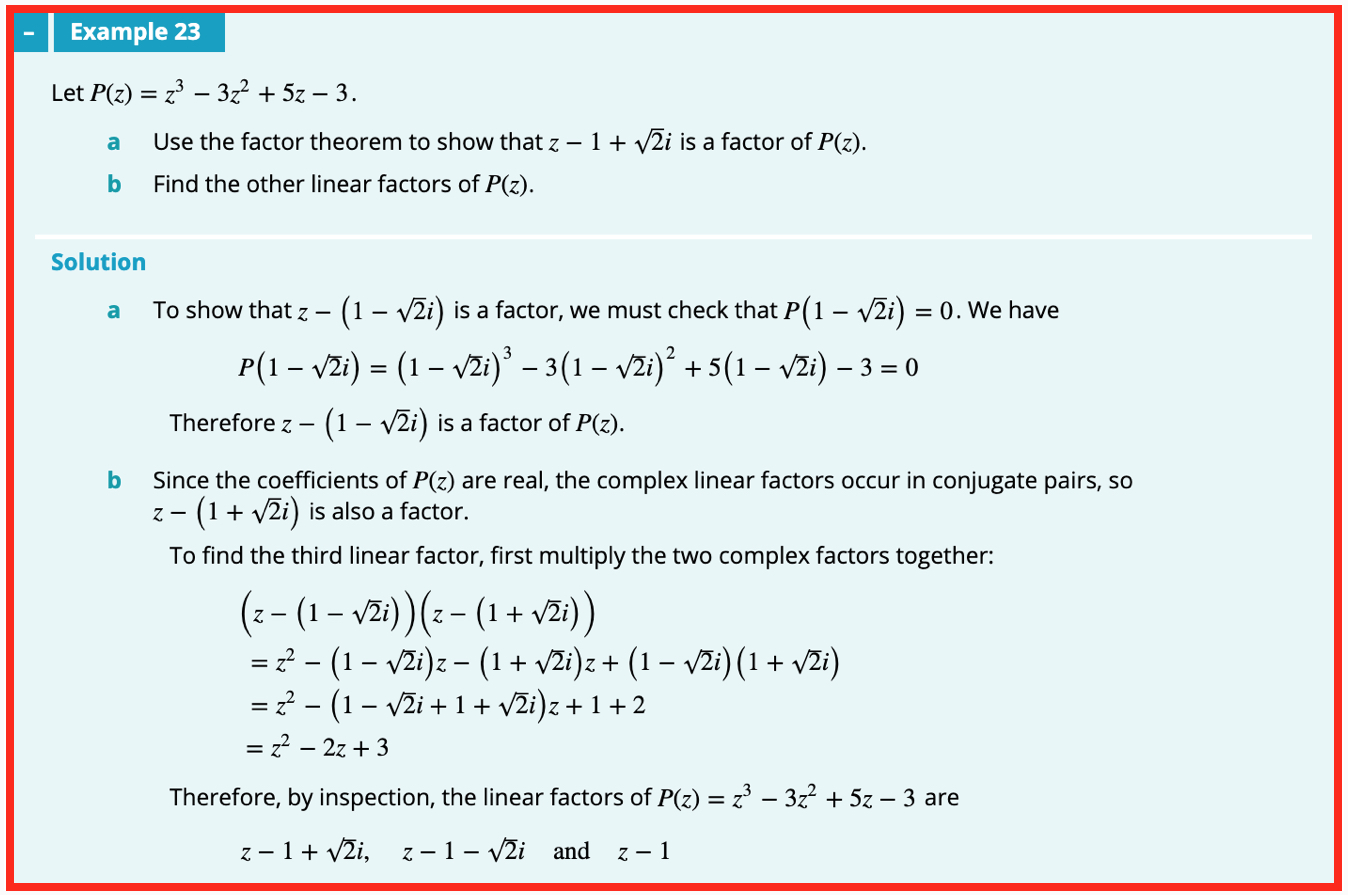# WitCH 16: The Root of Our Problem

This WitCH comes from one of our favourites, the Complex Numbers chapter from Cambridge’s Specialist Mathematics 3 & 4 (2019). It is not as deep or as beWitCHing as other aspects of the chapter. But, it’s still an impressive WitCH.## Update (11/08/19)

I guess if you’re gonna suggest a painful, ass-backwards method to solve a problem, you may as well fake the solution:

• Checking directly that P(1 – i√2) = 0 involves expanding a cubic, and more, which the text does in one single magic line.
• The painful multiplication of the products for part b is much more naturally and easily done as a difference of two squares: (z – 1 – i√2)(z – 1 + i√2) = (z – 1)2 + 2, etc.
• After all that the third factor, z – 1, is determined “by inspection”? Inspection of what?

AS RF notes, it is much easier to spot that z = 1 solves the cubic. Then some easy factoring (without long division …) gives P = (z – 1)(z2 – 2z + 3). Completing the square then leads to the linear factors, answering both parts of the question in the reverse, and natural, order.

Alternatively, as John notes, the difference of two squares calculation shows that if z – 1 + i√2 is a factor of P then so is the quadratic z^2 – 2z + 3. That this is so can then be checked (without long division …), giving P = (z – 1)(z^2 – 2z + 3), and so on, as before.

## 8 Replies to “WitCH 16: The Root of Our Problem”

1.Red Five says:

Surely any student serious enough about Mathematics to study Specialist can spot the z=1 solution almost immediately, from which the quadratic factor (and hence the two complex factors) can easily be found.

The whole thing just seems pointlessly over-complicated by a “show that” where the solution doesn’t really “show” anything. At least in the way VCAA would like (plus I’m sure VCAA would say the given solution is a “verify” rather than a “show that” which for some reason is different to them)

1.marty says:

Good point, RF. What if we stick to “method”, rather than spotting/guess? I do think the text solution “shows” what it claims.

2.Red Five says:

Really? The line underneath part (a) in the solution seems to make quite a jump…

1.marty says:

Oh, I see what you mean. Yes, of course you’re correct. It’s hilarious that they’ve just magically imagined the computation has been done. I just mean that their method was valid.

3.John says:

The expansion, in which an elephant gun is used to kill a fly, is the total crap in my book. Such an example should encourage recognition of a simple ‘sum of two squares’ factorised form:

([z – 1] – sqrti)([z – 1] + sqrti)

From which the irreducible quadratic can immediately immediately be written down by inspection. From which the remaining linear factor is easily constructed, giving the final root.

1.marty says:

Exactly, John. Using the factor theorem here is ludicrous. Related to your point, note the calculation in (b).

1.John says:

My comment was actually directly related to the expansion shown in part (b), but I agree using the factor theorem here is ludricous and deserves explicit comment. There are elephant guns everywhere in this example, not a fly swatter to be seen. It’s an ugly example.

How to remove all the crap? A much better example might have been:

Let P(z) = z^3 + az^2 + bz – 3.

Given that z – 1 + sqrti is a factor of P(z), find the values of a and b.

(In fairness to VCAA, I saw a question like my suggestion on Exam 1 a couple of years ago).

1.John says:

* where a and b are real numbers.

This site uses Akismet to reduce spam. Learn how your comment data is processed.